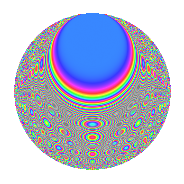# Properties

 Label 1008.2.xLevel 1008 Weight 2 Character orbit x Rep. character $$\chi_{1008}(307,\cdot)$$ Character field $$\Q(\zeta_{4})$$ Dimension 156 Sturm bound 384

# Related objects

## Defining parameters

 Level: $$N$$ = $$1008 = 2^{4} \cdot 3^{2} \cdot 7$$ Weight: $$k$$ = $$2$$ Character orbit: $$[\chi]$$ = 1008.x (of order $$4$$ and degree $$2$$) Character conductor: $$\operatorname{cond}(\chi)$$ = $$112$$ Character field: $$\Q(i)$$ Sturm bound: $$384$$

## Dimensions

The following table gives the dimensions of various subspaces of $$M_{2}(1008, [\chi])$$.

Total New Old
Modular forms 400 164 236
Cusp forms 368 156 212
Eisenstein series 32 8 24

## Trace form

 $$156q + 4q^{2} - 2q^{4} - 4q^{7} - 8q^{8} + O(q^{10})$$ $$156q + 4q^{2} - 2q^{4} - 4q^{7} - 8q^{8} + 14q^{14} - 6q^{16} - 26q^{22} + 16q^{23} - 8q^{28} - 4q^{29} + 24q^{32} - 20q^{35} - 12q^{37} - 22q^{44} + 8q^{46} - 4q^{49} + 22q^{50} - 4q^{53} + 50q^{56} - 6q^{58} - 50q^{64} + 8q^{65} - 32q^{67} + 32q^{70} + 72q^{71} + 62q^{74} + 16q^{77} + 16q^{85} - 62q^{86} + 38q^{88} - 28q^{91} - 48q^{92} + 4q^{98} + O(q^{100})$$

## Decomposition of $$S_{2}^{\mathrm{new}}(1008, [\chi])$$ into newform subspaces

The newforms in this space have not yet been added to the LMFDB.

## Decomposition of $$S_{2}^{\mathrm{old}}(1008, [\chi])$$ into lower level spaces

$$S_{2}^{\mathrm{old}}(1008, [\chi]) \cong$$ $$S_{2}^{\mathrm{new}}(112, [\chi])$$$$^{\oplus 3}$$$$\oplus$$$$S_{2}^{\mathrm{new}}(336, [\chi])$$$$^{\oplus 2}$$

## Hecke Characteristic Polynomials

There are no characteristic polynomials of Hecke operators in the database